The Central Limit Theorem

# 35 Using the Central Limit Theorem

### Examples of the Central Limit Theorem

#### Law of Large Numbers

The law of large numbers says that if you take samples of larger and larger size from any population, then the mean of the sampling distribution,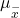tends to get closer and closer to the true population mean, μ. From the Central Limit Theorem, we know that as n gets larger and larger, the sample means follow a normal distribution. The larger n gets, the smaller the standard deviation of the sampling distribution gets. (Remember that the standard deviation for the sampling distribution of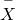is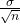.) This means that the sample mean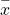must be closer to the population mean μ as n increases. We can say that μ is the value that the sample means approach as n gets larger. The Central Limit Theorem illustrates the law of large numbers.

This concept is so important and plays such a critical role in what follows it deserves to be developed further. Indeed, there are two critical issues that flow from the Central Limit Theorem and the application of the Law of Large numbers to it. These are

1. The probability density function of the sampling distribution of means is normally distributed regardless of the underlying distribution of the population observations and
2. standard deviation of the sampling distribution decreases as the size of the samples that were used to calculate the means for the sampling distribution increases.

Taking these in order. It would seem counterintuitive that the population may have any distribution and the distribution of means coming from it would be normally distributed. With the use of computers, experiments can be simulated that show the process by which the sampling distribution changes as the sample size is increased. These simulations show visually the results of the mathematical proof of the Central Limit Theorem.

Here are three examples of very different population distributions and the evolution of the sampling distribution to a normal distribution as the sample size increases. The top panel in these cases represents the histogram for the original data. The three panels show the histograms for 1,000 randomly drawn samples for different sample sizes: n=10, n= 25 and n=50. As the sample size increases, and the number of samples taken remains constant, the distribution of the 1,000 sample means becomes closer to the smooth line that represents the normal distribution.

(Figure) is for a normal distribution of individual observations and we would expect the sampling distribution to converge on the normal quickly. The results show this and show that even at a very small sample size the distribution is close to the normal distribution.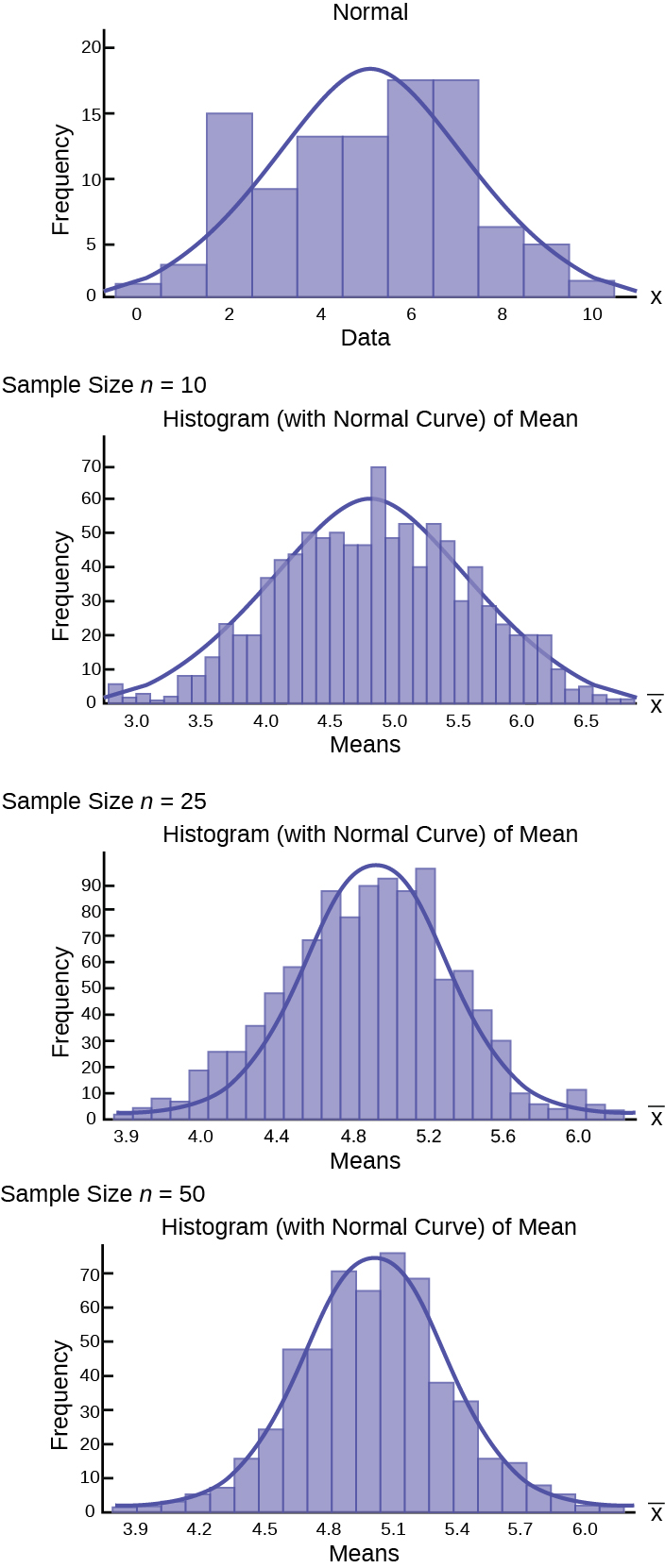(Figure) is a uniform distribution which, a bit amazingly, quickly approached the normal distribution even with only a sample of 10.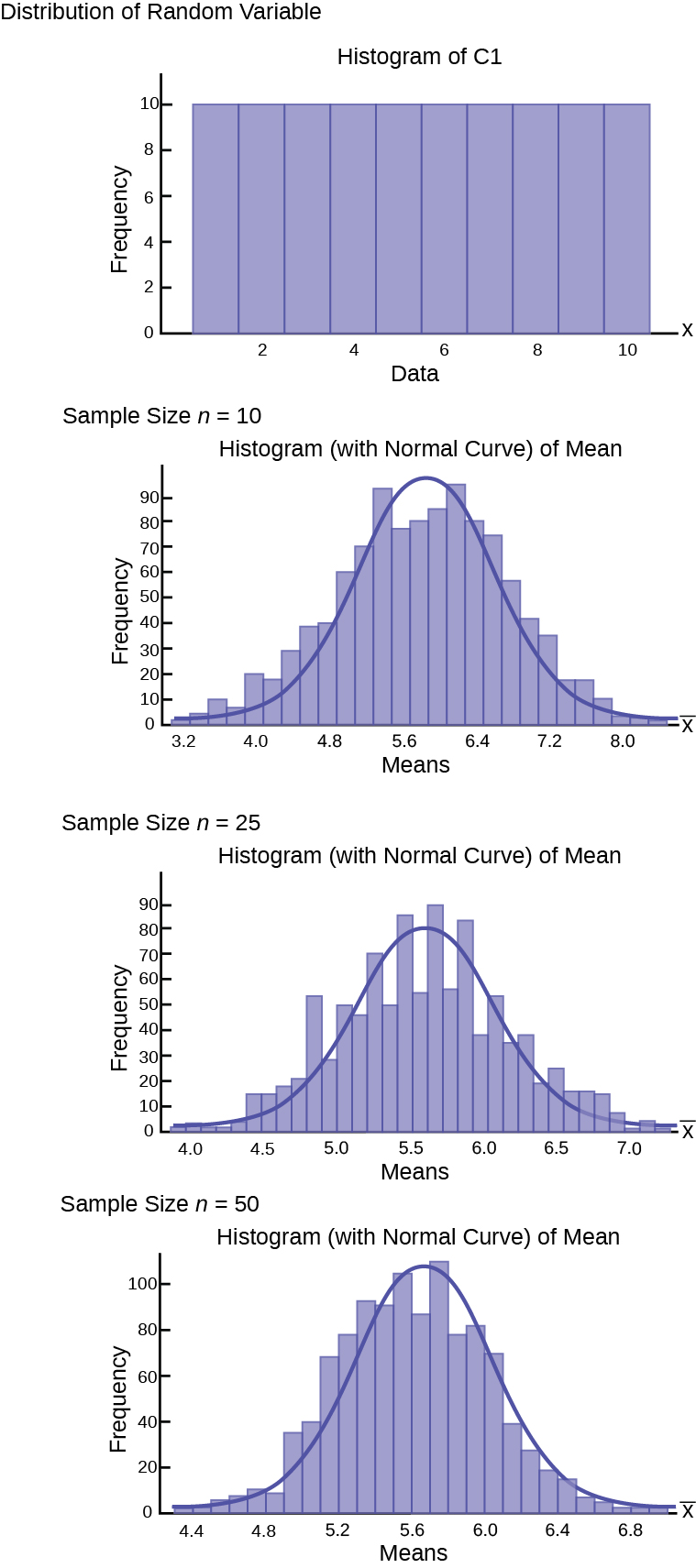(Figure) is a skewed distribution. This last one could be an exponential, geometric, or binomial with a small probability of success creating the skew in the distribution. For skewed distributions our intuition would say that this will take larger sample sizes to move to a normal distribution and indeed that is what we observe from the simulation. Nevertheless, at a sample size of 50, not considered a very large sample, the distribution of sample means has very decidedly gained the shape of the normal distribution.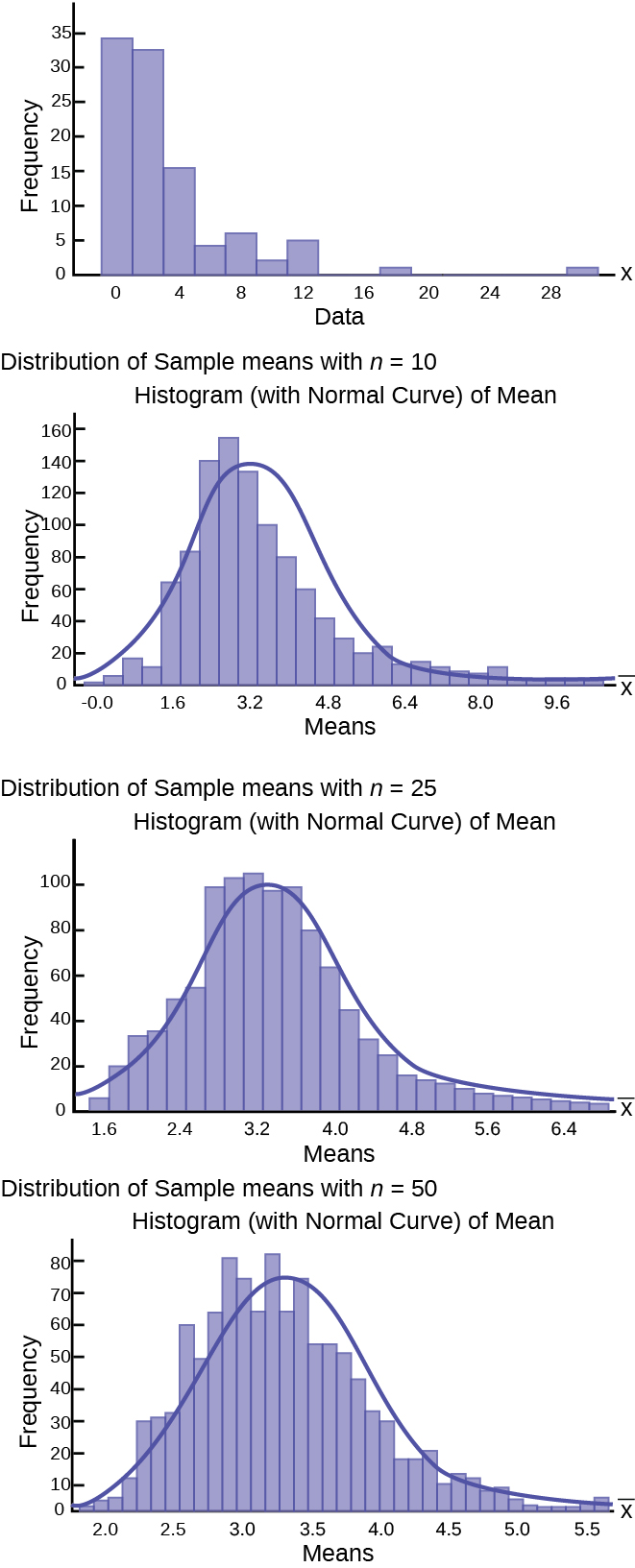The Central Limit Theorem provides more than the proof that the sampling distribution of means is normally distributed. It also provides us with the mean and standard deviation of this distribution. Further, as discussed above, the expected value of the mean,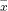, is equal to the mean of the population of the original data which is what we are interested in estimating from the sample we took. We have already inserted this conclusion of the Central Limit Theorem into the formula we use for standardizing from the sampling distribution to the standard normal distribution. And finally, the Central Limit Theorem has also provided the standard deviation of the sampling distribution,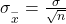, and this is critical to have to calculate probabilities of values of the new random variable,.

(Figure) shows a sampling distribution. The mean has been marked on the horizontal axis of the‘s and the standard deviation has been written to the right above the distribution. Notice that the standard deviation of the sampling distribution is the original standard deviation of the population, divided by the sample size. We have already seen that as the sample size increases the sampling distribution becomes closer and closer to the normal distribution. As this happens, the standard deviation of the sampling distribution changes in another way; the standard deviation decreases as n increases. At very very large n, the standard deviation of the sampling distribution becomes very small and at infinity it collapses on top of the population mean. This is what it means that the expected value of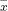is the population mean, µ.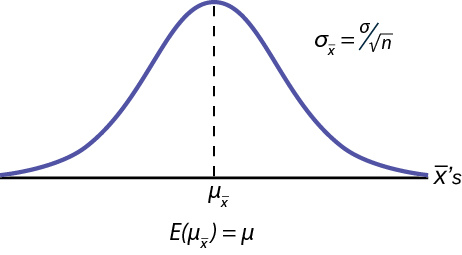At non-extreme values of n,this relationship between the standard deviation of the sampling distribution and the sample size plays a very important part in our ability to estimate the parameters we are interested in.

(Figure) shows three sampling distributions. The only change that was made is the sample size that was used to get the sample means for each distribution. As the sample size increases, n goes from 10 to 30 to 50, the standard deviations of the respective sampling distributions decrease because the sample size is in the denominator of the standard deviations of the sampling distributions.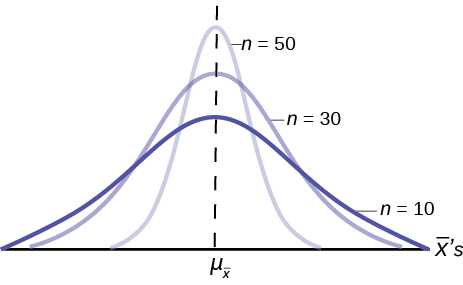The implications for this are very important. (Figure) shows the effect of the sample size on the confidence we will have in our estimates. These are two sampling distributions from the same population. One sampling distribution was created with samples of size 10 and the other with samples of size 50. All other things constant, the sampling distribution with sample size 50 has a smaller standard deviation that causes the graph to be higher and narrower. The important effect of this is that for the same probability of one standard deviation from the mean, this distribution covers much less of a range of possible values than the other distribution. One standard deviation is marked on theaxis for each distribution. This is shown by the two arrows that are plus or minus one standard deviation for each distribution. If the probability that the true mean is one standard deviation away from the mean, then for the sampling distribution with the smaller sample size, the possible range of values is much greater. A simple question is, would you rather have a sample mean from the narrow, tight distribution, or the flat, wide distribution as the estimate of the population mean? Your answer tells us why people intuitively will always choose data from a large sample rather than a small sample. The sample mean they are getting is coming from a more compact distribution. This concept will be the foundation for what will be called level of confidence in the next unit.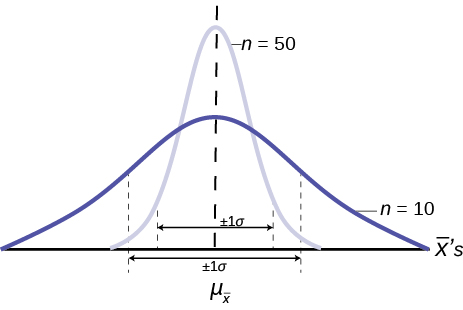### Chapter Review

The Central Limit Theorem can be used to illustrate the law of large numbers. The law of large numbers states that the larger the sample size you take from a population, the closer the sample meangets to μ.

Use the following information to answer the next ten exercises: A manufacturer produces 25-pound lifting weights. The lowest actual weight is 24 pounds, and the highest is 26 pounds. Each weight is equally likely so the distribution of weights is uniform. A sample of 100 weights is taken.

1. What is the distribution for the weights of one 25-pound lifting weight? What is the mean and standard deivation?
2. What is the distribution for the mean weight of 100 25-pound lifting weights?
3. Find the probability that the mean actual weight for the 100 weights is less than 24.9.
1. U(24, 26), 25, 0.5774
2. N(25, 0.0577)
3. 0.0416

Draw the graph from (Figure)

<!– <solution id=”fs-idm124774096″>

–>

Find the probability that the mean actual weight for the 100 weights is greater than 25.2.

0.0003

Draw the graph from (Figure)

<!– <solution id=”fs-idm90694544″>

–>

Find the 90th percentile for the mean weight for the 100 weights.

25.07

Draw the graph from (Figure)

<!– <solution id=”fs-idm110267328″>

–>

1. What is the distribution for the sum of the weights of 100 25-pound lifting weights?
2. Find P(Σx < 2,450).
1. N(2,500, 5.7735)
2. 0

Draw the graph from (Figure)

<!– <solution id=”fs-idp11678144″>

–>

Find the 90th percentile for the total weight of the 100 weights.

2,507.40

Draw the graph from (Figure)

<!– <solution id=”fs-idm17187952″>

–>

Use the following information to answer the next five exercises:
The length of time a particular smartphone’s battery lasts follows an exponential distribution with a mean of ten months. A sample of 64 of these smartphones is taken.

1. What is the standard deviation?
2. What is the parameter m?
1. 10
2.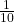What is the distribution for the length of time one battery lasts?

<!– <solution id=”fs-idm75203552″> Exp ( 1 10 ) –>

What is the distribution for the mean length of time 64 batteries last?

N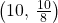What is the distribution for the total length of time 64 batteries last?

<!– <solution id=”fs-idm141956784″> N(640, 80) –>

Find the probability that the sample mean is between seven and 11.

0.7799

Find the 80th percentile for the total length of time 64 batteries last.

<!– <solution id=”fs-idm4580720″>707.3 –>

Find the IQR for the mean amount of time 64 batteries last.

1.69

Find the middle 80% for the total amount of time 64 batteries last.

<!– <solution id=”fs-idm72677136″>205.05 –>

Use the following information to answer the next eight exercises:
A uniform distribution has a minimum of six and a maximum of ten. A sample of 50 is taken.

Find P(Σx > 420).

0.0072

Find the 90th percentile for the sums.

<!– <solution id=”fs-idm96012160″> 410.46 –>

Find the 15th percentile for the sums.

391.54

Find the first quartile for the sums.

<!– <solution id=”fs-idp5340032″>394.49 –>

Find the third quartile for the sums.

405.51

Find the 80th percentile for the sums.

<!– <solution id=”fs-idm3823936″>406.87 –>

A population has a mean of 25 and a standard deviation of 2. If it is sampled repeatedly with samples of size 49, what is the mean and standard deviation of the sample means?

Mean = 25, standard deviation = 2/7

A population has a mean of 48 and a standard deviation of 5. If it is sampled repeatedly with samples of size 36, what is the mean and standard deviation of the sample means?

Mean = 48, standard deviation = 5/6

A population has a mean of 90 and a standard deviation of 6. If it is sampled repeatedly with samples of size 64, what is the mean and standard deviation of the sample means?

Mean = 90, standard deviation = 3/4

A population has a mean of 120 and a standard deviation of 2.4. If it is sampled repeatedly with samples of size 40, what is the mean and standard deviation of the sample means?

Mean = 120, standard deviation = 0.38

A population has a mean of 17 and a standard deviation of 1.2. If it is sampled repeatedly with samples of size 50, what is the mean and standard deviation of the sample means?

Mean = 17, standard deviation = 0.17

A population has a mean of 17 and a standard deviation of 0.2. If it is sampled repeatedly with samples of size 16, what is the expected value and standard deviation of the sample means?

Expected value = 17, standard deviation = 0.05

A population has a mean of 38 and a standard deviation of 3. If it is sampled repeatedly with samples of size 48, what is the expected value and standard deviation of the sample means?

Expected value = 38, standard deviation = 0.43

A population has a mean of 14 and a standard deviation of 5. If it is sampled repeatedly with samples of size 60, what is the expected value and standard deviation of the sample means?

Expected value = 14, standard deviation = 0.65

#### Homework

A large population of 5,000 students take a practice test to prepare for a standardized test. The population mean is 140 questions correct, and the standard deviation is 80. What size samples should a researcher take to get a distribution of means of the samples with a standard deviation of 10?

64

A large population has skewed data with a mean of 70 and a standard deviation of 6. Samples of size 100 are taken, and the distribution of the means of these samples is analyzed.

1. Will the distribution of the means be closer to a normal distribution than the distribution of the population?
2. Will the mean of the means of the samples remain close to 70?
3. Will the distribution of the means have a smaller standard deviation?
4. What is that standard deviation?
1. Yes
2. Yes
3. Yes
4. 0.6

A researcher is looking at data from a large population with a standard deviation that is much too large. In order to concentrate the information, the researcher decides to repeatedly sample the data and use the distribution of the means of the samples? The first effort used sample sized of 100. But the standard deviation was about double the value the researcher wanted. What is the smallest size samples the researcher can use to remedy the problem?

400

A researcher looks at a large set of data, and concludes the population has a standard deviation of 40. Using sample sizes of 64, the researcher is able to focus the mean of the means of the sample to a narrower distribution where the standard deviation is 5. Then, the researcher realizes there was an error in the original calculations, and the initial standard deviation is really 20. Since the standard deviation of the means of the samples was obtained using the original standard deviation, this value is also impacted by the discovery of the error. What is the correct value of the standard deviation of the means of the samples?

2.5

A population has a standard deviation of 50. It is sampled with samples of size 100. What is the variance of the means of the samples?

25

### Key Terms

Mean
a number that measures the central tendency; a common name for mean is “average.” The term “mean” is a shortened form of “arithmetic mean.” By definition, the mean for a sample (denoted by) is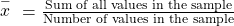, and the mean for a population (denoted by μ) is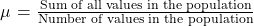.
Finite Population Correction Factor
adjusts the variance of the sampling distribution if the population is known and more than 5% of the population is being sampled.
Normal Distribution
a continuous random variable with pdf, where μ is the mean of the distribution and σ is the standard deviation.; notation: X ~ N(μ, σ). If μ = 0 and σ = 1, the random variable, Z, is called the standard normal distribution.
Standard Error of the Proportion
the standard deviation of the sampling distribution of proportions